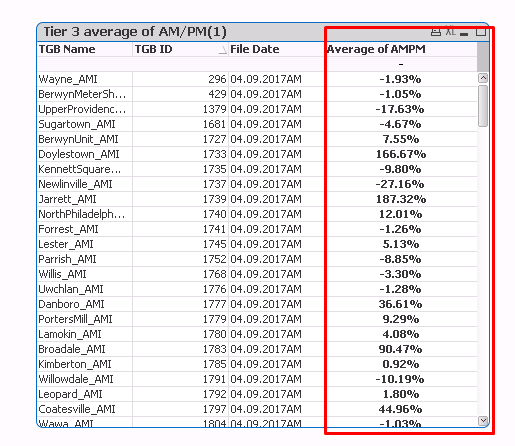# QlikView App Development

Discussion Board for collaboration related to QlikView App Development.

HighlightedNot applicable

## how to change the color of the values in straight table?

how to change the color of the values in the straight table?

Requirements:

1. if the Average of AM and PM Goes More than - 90 % means the value need to be shown in Red Color.

2. if the Average of AM and PM values is between -50% to -89%  means the value need to be shown in Blue Color

kindly help me so that it will be help full for my learning Process

Straight table:Expression:Thanks,

Muthu

7 Replies
Valued Contributor

## Re: how to change the color of the values in straight table?

Hi

Go to Expression tab--> Click on Expression left side of Plus(+) symbal----> Click on Text Color

write the condition as per your requirement

Valued Contributor III

## Re: how to change the color of the values in straight table?

Lets Assume your expression is Sum(Sales),

goto the expression tab and Click '+' symbol below your expression and

write the following code as

if(Sum(Sales)>9000 , Red(),Blue())

Valued Contributor III

## Re: how to change the color of the values in straight table?

You need to write this expression in "Text Color" Missed thatNot applicable

## Re: how to change the color of the values in straight table?

Go to Visual cues tab in straight table

and specify the lower and upper values as per ur requirementNot applicable

## Re: how to change the color of the values in straight table?

Visual Cues

select your expression(in case if you have multiple) and give the color based on the range '>=',Normal, '<='

New Contributor III

## Re: how to change the color of the values in straight table?

To be able to give a better answer based on your dataset, can you create a sample Qlik QVD file containing data samples (as you state in your screenshots.

Coloring of the specific column using color functions can be checked in the attached file.
You can create an if statement to check for the values needed. If multiple colors are used/needed, nest IF statements.Remember that when working with percentages you need to check for values between -1.0 and +1.0 (assuming the value is never above 100%)

The if construction might be something like the following:

= if(num(IF(AMPM = 'AM',

SUM({<AMPM = {PM}>} TOTAL <[TBG Name],Filedate> count)/SUM({<AMPM = {AM}>} TOTAL <[TBG ID]> count)-1,

SUM({<AMPM = {AM}>} TOTAL <[TBG Name],Filedate> count)/SUM({<AMPM = {PM}>} TOTAL <[TBG ID]> count)-1

)

)<-.90, rgb(234,0,0),

if(num(IF(AMPM = 'AM',

SUM({<AMPM = {PM}>} TOTAL <[TBG Name],Filedate> count)/SUM({<AMPM = {AM}>} TOTAL <[TBG ID]> count)-1,

SUM({<AMPM = {AM}>} TOTAL <[TBG Name],Filedate> count)/SUM({<AMPM = {PM}>} TOTAL <[TBG ID]> count)-1

)

)<-.75

,rgb(0,0,234),

rgb(0,234,0)

)

)

regards,

Ferry

Contributor

## Re: how to change the color of the values in straight table?

try this: in text color of expression

=If([Average of AM and PM]<=-90,Red(),If([Average of AM and PM]<=-50 and [Average of AM and PM]>=-89,Blue()))

instead of [Average of AM and PM] field  in my formula ,you should copy of all your expression (num (if(rolling _7 ....))) or define a variable for [Average of AM and PM] and call it in definition part of Text color for your expression